﻿ 近浅海条件下被动声呐浮标使用深度分析与研究
 舰船科学技术2018, Vol. 40Issue (3): 128-131PDF

Analysis and research on the depth of passive sonobuoy in shallow sea
ZHANG Fei-fei, ZHAO Shen-dong, LIU Zhao-hui, LIANG Zuo-tang
Qingdao Branch of Naval Aeronautical Engineering Academy, Qingdao 266041, China
Abstract: The detection range of passive sonobuoy is related to not only the sound speed profile, but also the submarine target characteristic and the depth of sonobuoy in the water. This study analyzes the effect of these three kinds of typical sound speed profiles to acoustic propagation characteristic by the BELLHOP model. And it talks about the effect of the depth of passive sonobuoy to the detection range when submarine is in different depth. The result shows that the detection range is different if the depth of sonobuoy is different. The performance of sonobuoy can be well exerted and the targets of different depth can be detected well when finding the optimum detection depth of different sound speed profiles.
Key words: shallow sea     passive sonobuoy     sound speed profile     submarine target characteristic
0 引　言

1 潜艇辐射噪声模型

 ${V_c} = {V_{c100}}\sqrt {\frac{{p + \rho gH - p_d^{}}}{{p + 100\rho g - {p_{d100}}}}} \text{，}$ (1)

 $SL = \left\{ \begin{array}{l}25\lg {V_m} + 77 + 20a\;\;\text{，}\;\;\;\;\;\;\;\;\;\;\;{V_m} < {V_c}\text{，}\\104 + 20a + \Delta \;\;\text{，}\;\;\;\;\;\;\;\;\;\;\;\;\;\;\;\;\;\;{V_m} = {V_c}\text{，}\\104 + 20a + \Delta + b({V_m} - {V_c})\;\;\text{，}\;{V_m} > {V_c}\text{。}\end{array} \right.$ (2)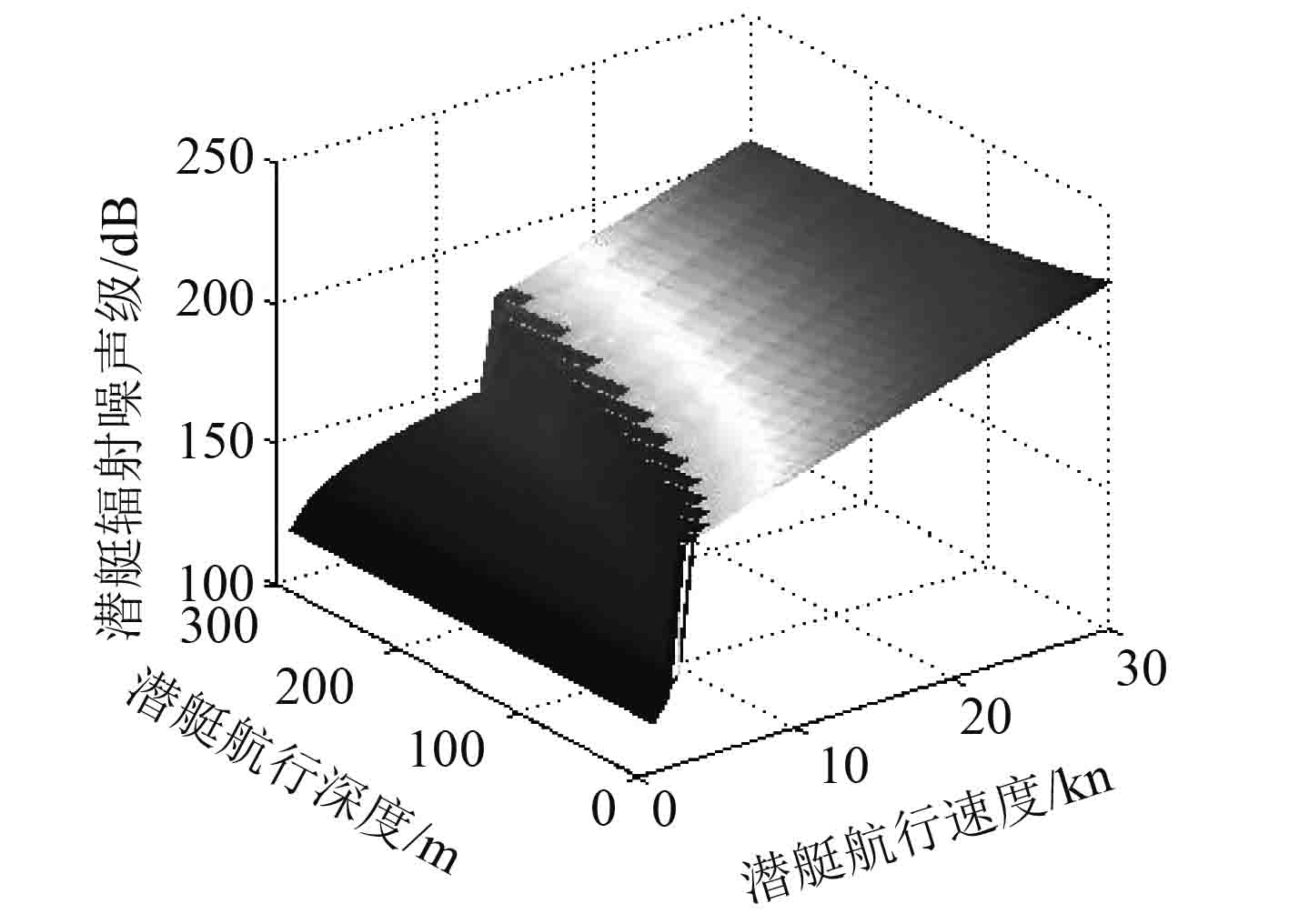图 1 潜艇的辐射噪声与航深和航度的关系 Fig. 1 The relationship of the submarine’s radiated noise and the depth and speed
2 海洋环境噪声模型

 $N{L_f} = 10\lg {f^{ - 1.7}} + 6S + 55\text{，}$ (3)

 $\begin{split}NL = 10\lg \left( {\int_{{f_1}}^{{f_2}} {{{10}^{\frac{{N{L_f}}}{{10}}}}{ d}f} } \right) =& 10\lg \left( {f_1^{ - 0.7} - f_2^{ - 0.7}} \right) + \\& 6S + 56.5\text{。}\end{split}$ (4)
3 基于射线理论的浅海传播损失计算方法

 $p\left( {s,n} \right) = A\left( s \right)\phi \left( {s,n} \right){e^{j\omega \tau }}\text{。}$ (5)

 $\begin{split}& \displaystyle\frac{{{ d}r}}{{{ d}s}} = c\xi \left( s \right),\;\displaystyle\frac{{{ d}\xi }}{{{ d}s}} = - \frac{1}{{{c^2}}}\frac{{{ d}c}}{{{ d}r}}\text{，}\\& \displaystyle\frac{{{ d}z}}{{{ d}s}} = c\zeta \left( s \right),\;\displaystyle\frac{{{ d}\zeta }}{{{ d}s}} = - \frac{1}{{{c^2}}}\frac{{{ d}c}}{{{ d}z}}\text{。}\end{split}$ (6)

 $\frac{{{ d}v}}{{{ d}s}} = cu\left( s \right),\;\frac{{{ d}u}}{{{ d}s}} = - \frac{{{c_{nn}}}}{{{c^2}\left( s \right)}}v\left( s \right)\text{。}$ (7)

 $\phi \left( {n,s} \right) = \exp \left[ { - {{\left( {n/W} \right)}^2}} \right]\text{，}$ (8)
 $A\left( s \right) = \frac{1}{{{{\left( {2\pi } \right)}^{1/4}}}}\sqrt {\frac{c}{{c\left( 0 \right)}}\frac{{\delta \alpha }}{r}\frac{{2\cos \alpha }}{W}} \text{，}$ (9)
 $W = v\left( s \right)\delta \alpha /c\left( 0 \right)\text{。}$ (10)

 $\tau \left( s \right) = \tau \left( 0 \right) = \int_0^S {\frac{1}{{c\left( {s'} \right)}}{ d}s'} \text{。}$ (11)

${p_j}\left( {s,n} \right)$ 转化为柱坐标系下的声压 ${p_j}\left( {r,z} \right)$ ，由携带不同能量声线的叠加得到最终的声场。本文采用相干声压场的计算方法，声压pc为：

 ${p_c}\left( {r,z} \right) = \sum\limits_{j = 1}^N {{p_j}\left( {r,z} \right)} \text{。}$ (12)

4 典型声速剖面下的声传播及声传播损失 4.1 正梯度声速剖面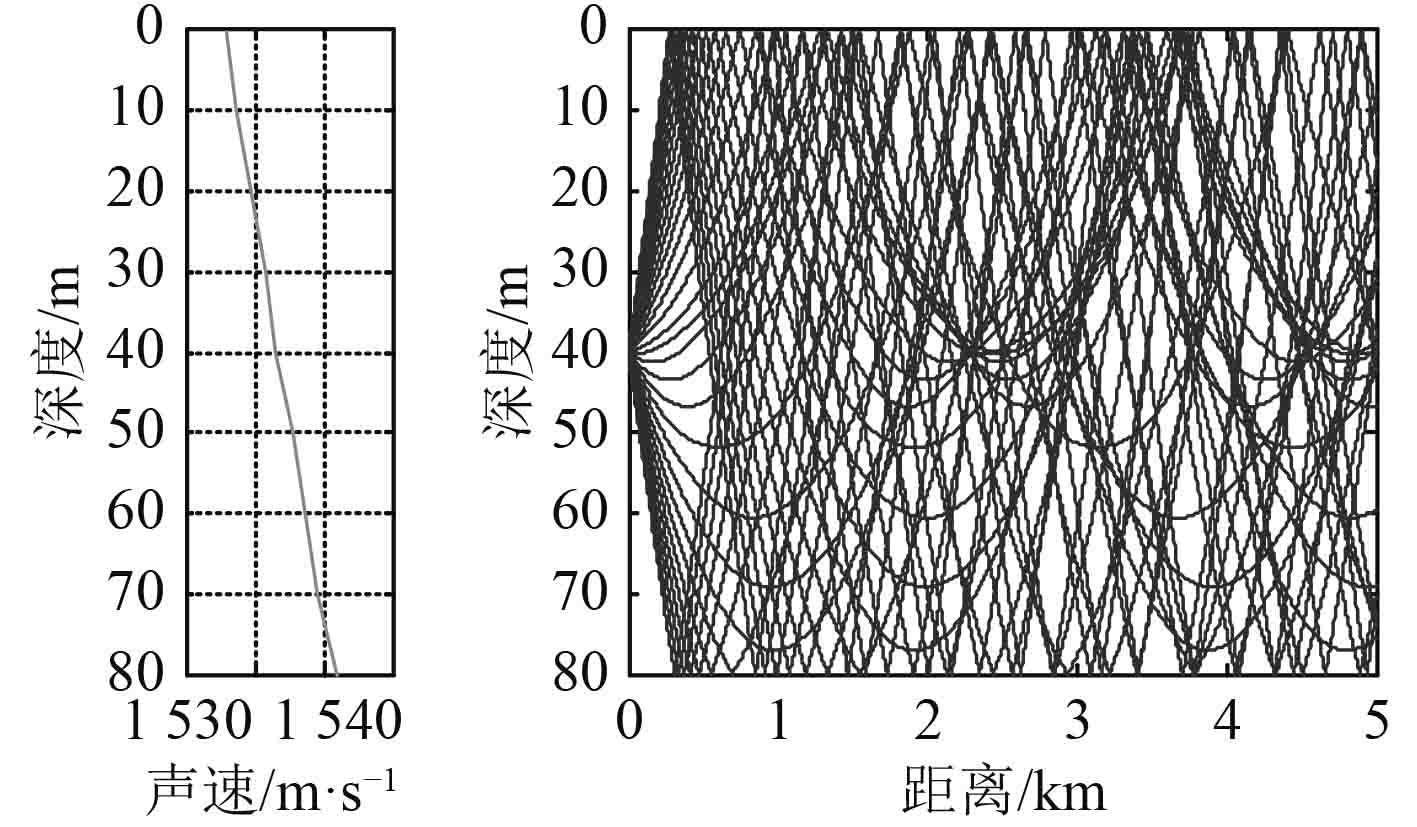图 2 正梯度声速剖面时的声传播特性 Fig. 2 Acoustic propagation characteristic existing positive gradient sound speed profile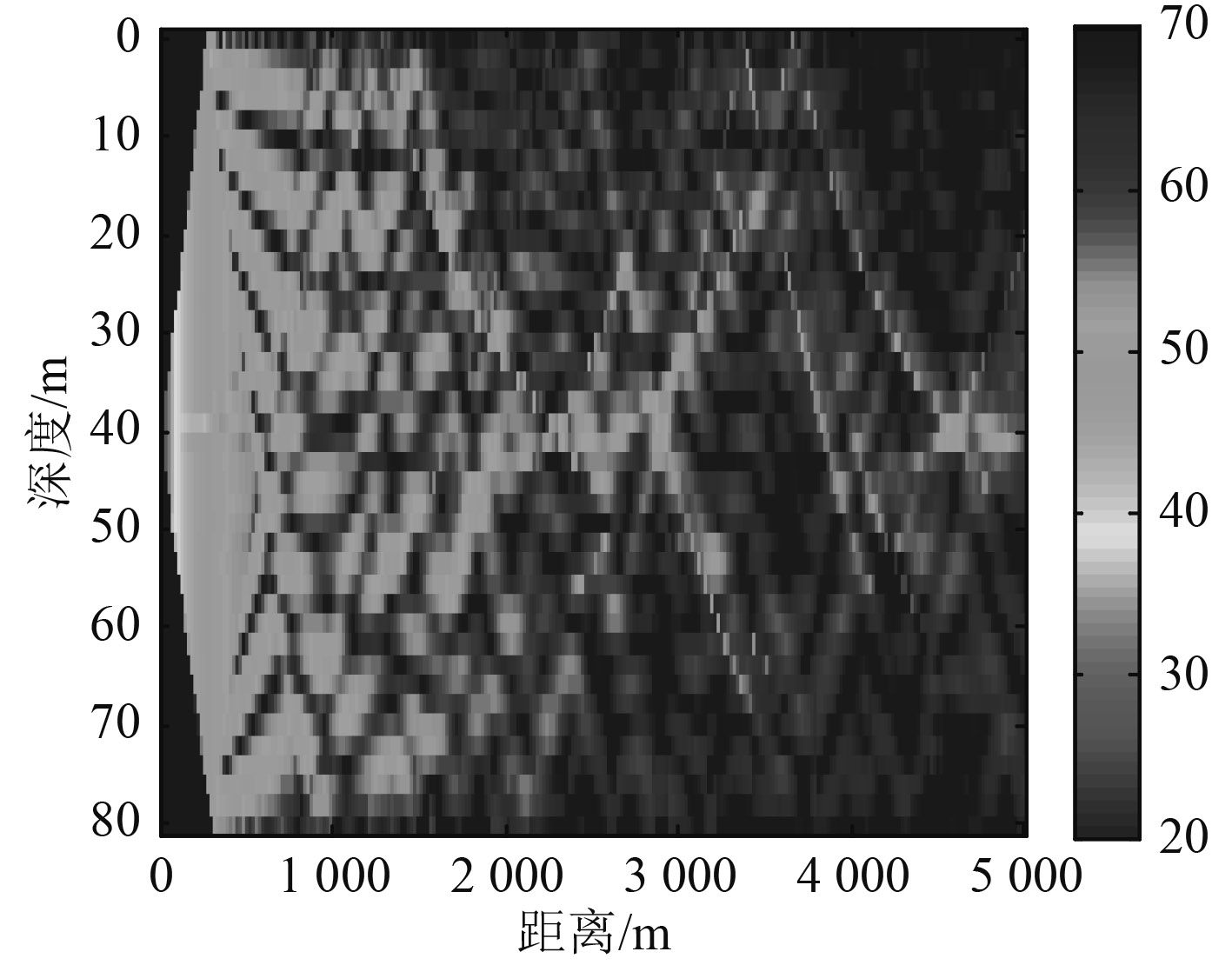图 3 正梯度声速剖面时的声传播损失 Fig. 3 Transmission loss existing positive gradient sound speed profile
4.2 负梯度声速剖面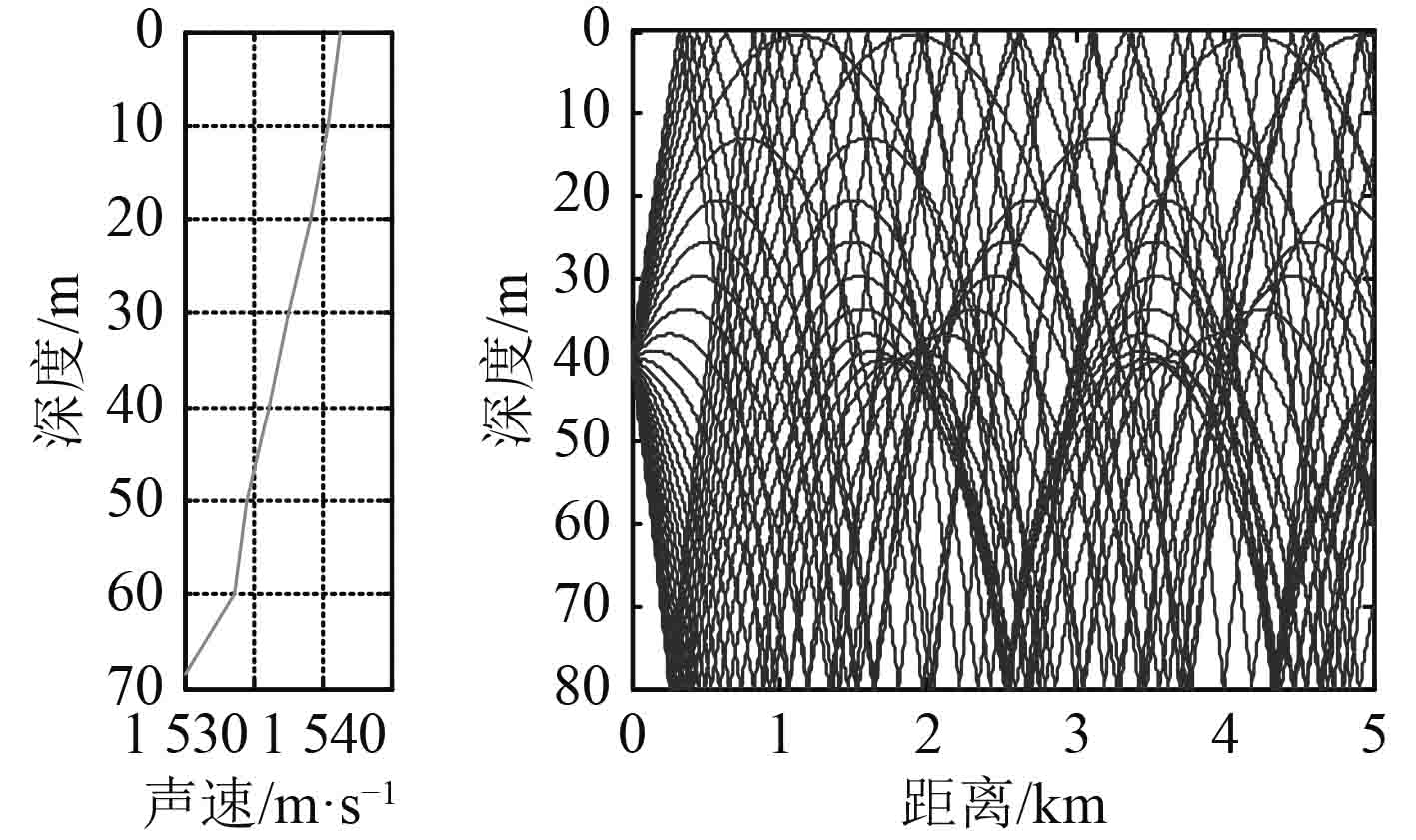图 4 负梯度声速剖面时的声传播特性 Fig. 4 Acoustic propagation characteristic existing negative gradient sound speed profile图 5 负梯度声速剖面时的声传播损失 Fig. 5 Transmission loss existing negative gradient sound speed profile
4.3 反声道声速剖面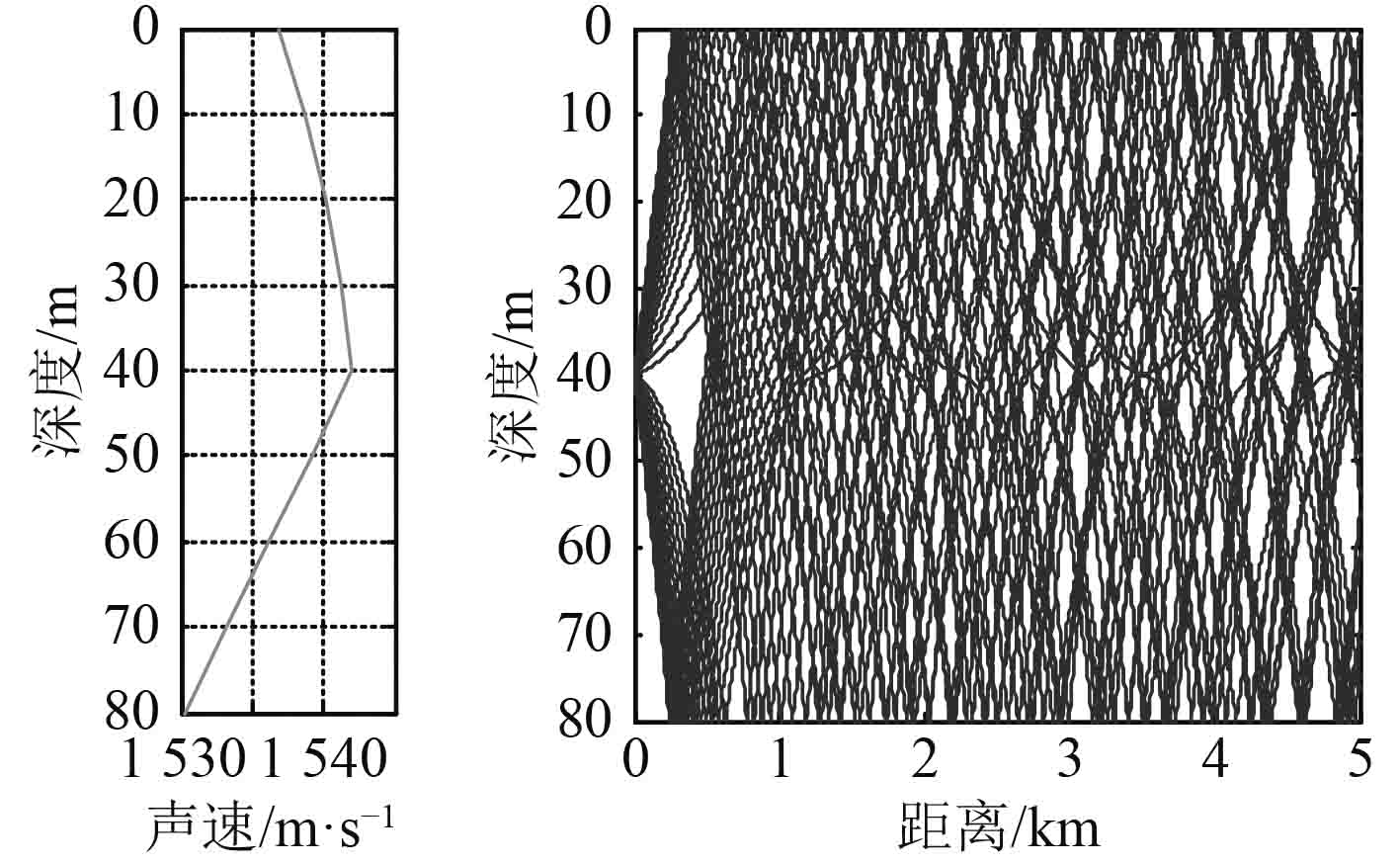图 6 反声道声速剖面时的声传播特性 Fig. 6 Acoustic propagation characteristic existing negative sound channel sound speed profile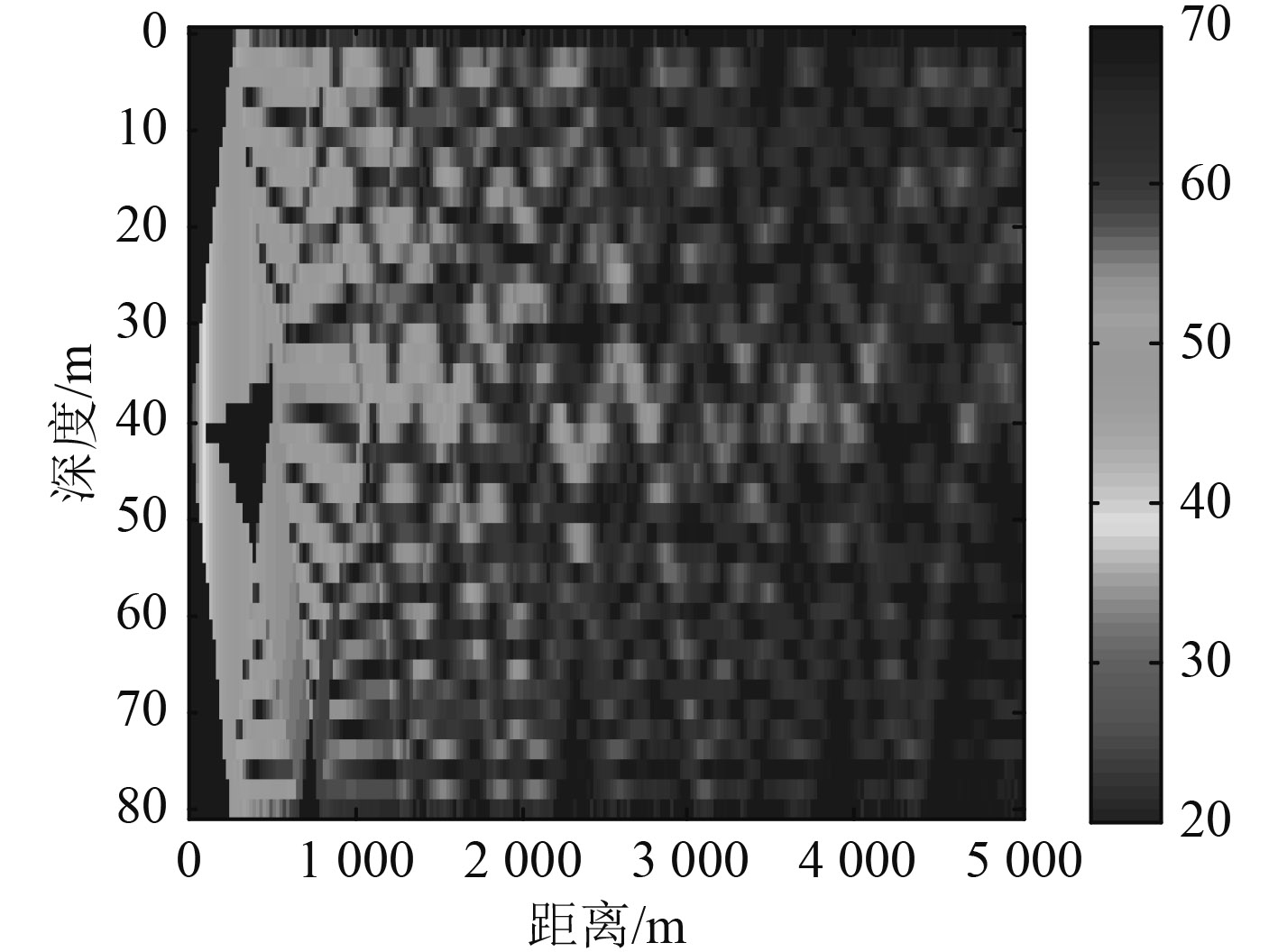图 7 反声道声速剖面时的声传播损失 Fig. 7 Transmission loss existing negative sound channel sound speed profile
5 被动声呐浮标的探测距离仿真分析

 $SE = SL - NL - TL - DT + DI\text{，}$ (13)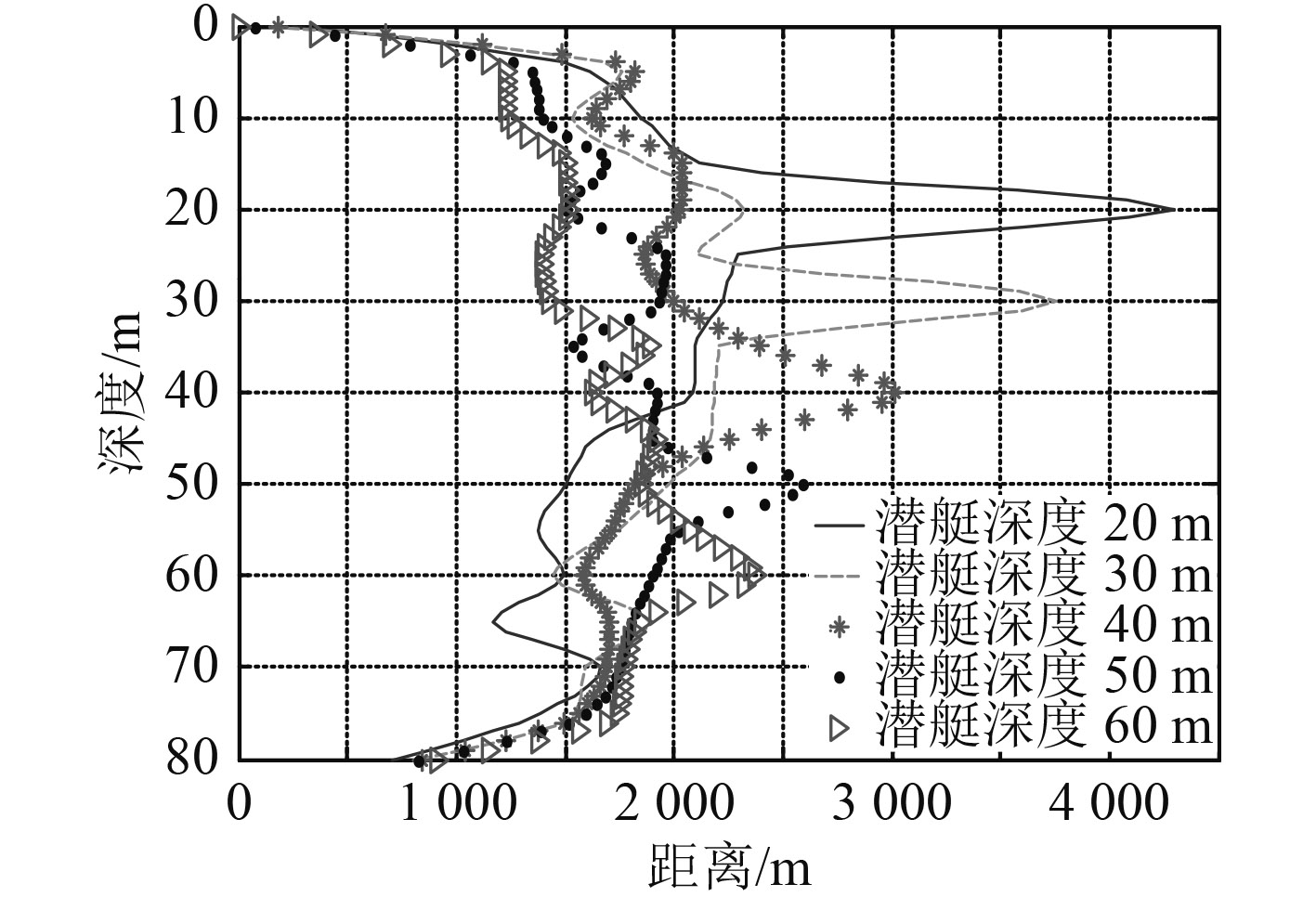图 8 正梯度声速剖面下被动声呐浮标探测距离 Fig. 8 The detection range of passive sonobuoy when positive gradient sound speed profile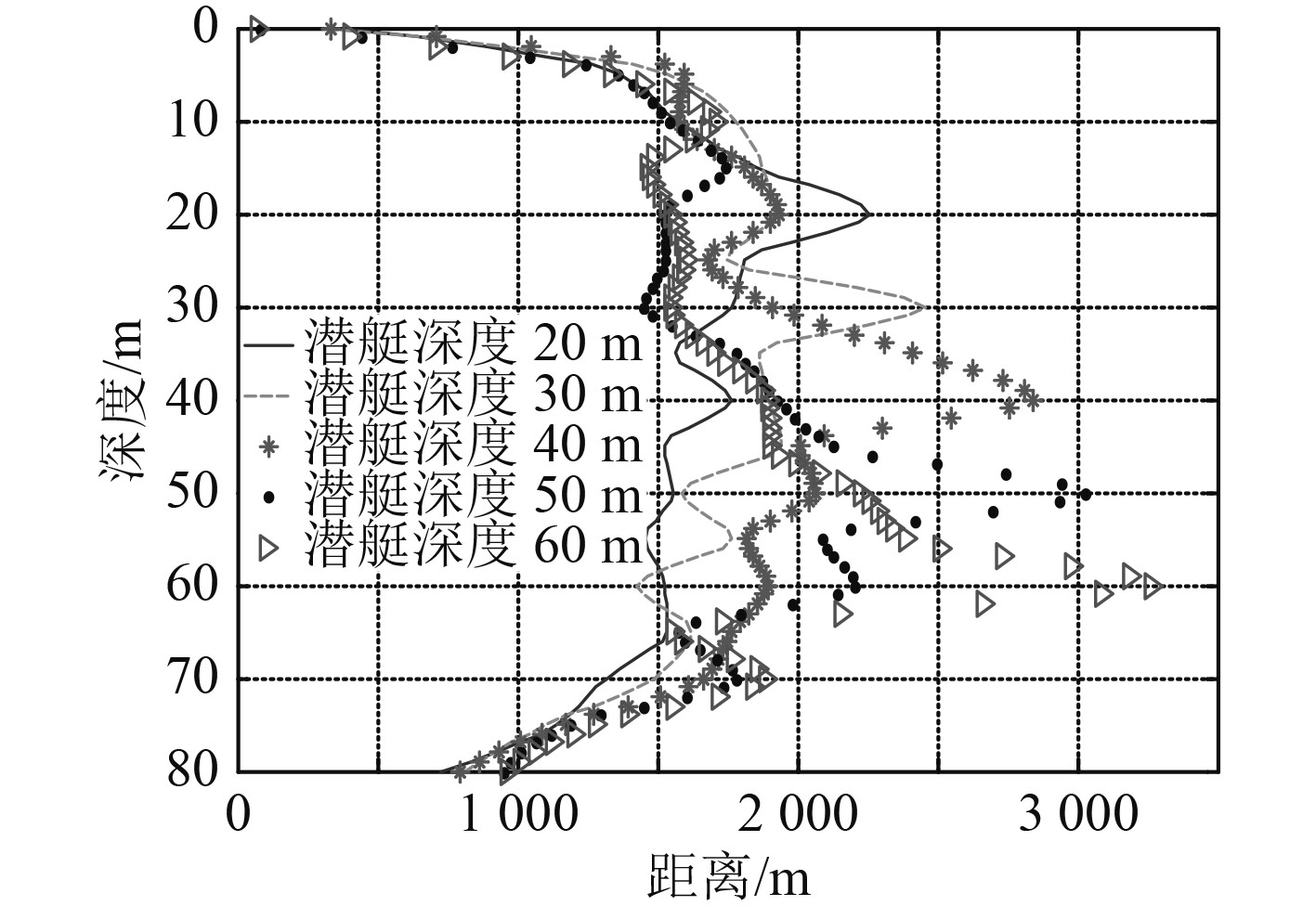图 9 负梯度声速剖面下被动声呐浮标探测距离 Fig. 9 The detection range of passive sonobuoy when negative gradient sound speed profile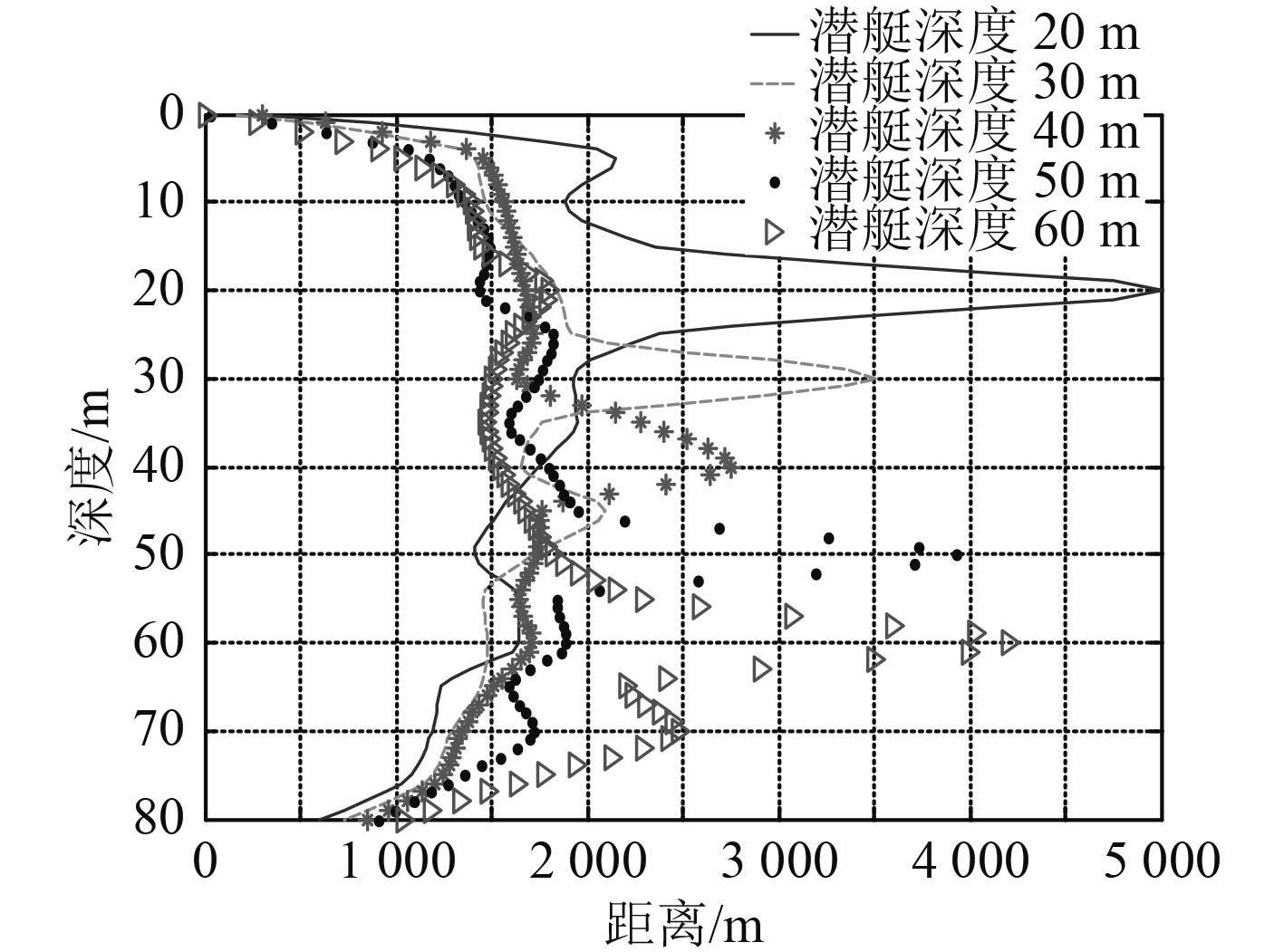图 10 反声道声速剖面下被动声呐浮标探测距离 Fig. 10 The detection range of passive sonobuoy when negative sound channel sound speed profile

6 结　语

  张纪铃, 胡鹏涛. 浅海声速剖面对声呐作用距离的影响研究[J]. 电声技术, 2014, 38(10): 36–38.  高守勇, 王升, 马力, 等. 潜艇辐射噪声近场特性研究[J]. 声学技术, 2011, 30(3): 7–9. http://industry.wanfangdata.com.cn/dl/Detail/Thesis?id=Thesis_Y936989  高学强, 杨日杰. 潜艇辐射噪声声源级经验公式修正[J]. 声学与电子工程, 2007, 87: 17–18. https://www.wenkuxiazai.com/doc/1e5ef1e27c1cfad6195fa7cf.html  刘伯胜, 雷家煜. 水声学原理[M]. 哈尔滨: 哈尔滨工程大学出版社, 2010.  孟春霞, 李桂娟, 张 亮. 浅海环境噪声垂直指向性获取与分析[J]. 声学技术, 2013, 32(5): 37–39. http://www.cnki.com.cn/Article/CJFDTotal-SCXH197710009.htm  刘盂庵, 连立民. 水声工程[M]. 杭州: 浙江科学技术出版社, 2002: 72–73.  梁民赞, 孟华, 陈迎春, 等. 水声环境复杂性对声呐探测距离的影响[J]. 船舶科学技术, 2013, 35(4): 45–48. http://wuxizazhi.cnki.net/Sub/wqjs/a/JCKX201304012.html